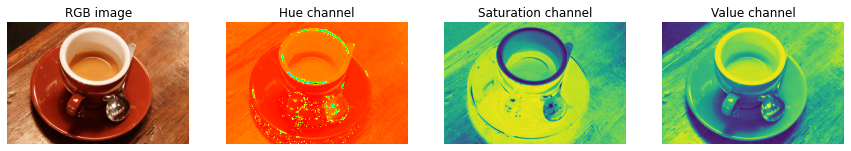# 20. What’s this notebook about?¶

This example illustrates how RGB to HSV (Hue, Saturation, Value) conversion can be used to facilitate segmentation processes.

Usually, objects in images have distinct colors (hues) and luminosities, so that these features can be used to separate different areas of the image. In the RGB representation the hue and the luminosity are expressed as a linear combination of the R,G,B channels, whereas they correspond to single channels of the HSV image (the Hue and the Value channels).

A simple segmentation of the image can then be effectively performed by a mere thresholding of the HSV channels.

See: https://en.wikipedia.org/wiki/HSL_and_HSV

import matplotlib.pyplot as plt

from skimage import data
from skimage.color import rgb2hsv


## 20.1. Original and HSV converted image (using rgb2hsv() method)¶

rgb_img = data.coffee()
hsv_img = rgb2hsv(rgb_img)


## 20.2. Images with hue, saturation, and value channels (separately)¶

hue_img = hsv_img[:, :, 0]
sat_img = hsv_img[:, :, 1]
value_img = hsv_img[:, :, 2]


## 20.3. Show images¶

fig, (ax0, ax1, ax2, ax3) = plt.subplots(ncols=4, figsize=(15, 5))

ax0.imshow(rgb_img)
ax0.set_title("RGB image")
ax0.axis('off')

ax1.imshow(hue_img, cmap='hsv')
ax1.set_title("Hue channel")
ax1.axis('off')

ax2.imshow(sat_img)
ax2.set_title("Saturation channel")
ax2.axis('off')

ax3.imshow(value_img)
ax3.set_title("Value channel")
ax3.axis('off')

plt.show()